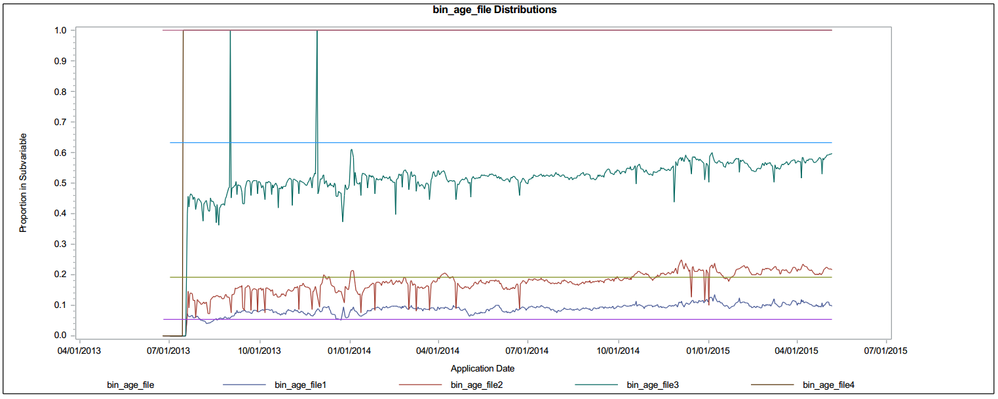## Controlling Line Color in proc gplot for Two by Groups

I am plotting two by groups of the form y*x=z overlaid on the same plot. SGPlot is perfect for handling grouping but not groups of groups. I have incoming empirical data that we are using to monitor baseline assumptions. This creates a signal and a straight line baseline across a number of variables. I do not need a legend for the baseline variables. I have successfully created the desired plot by using two plot statements in proc gplot and then suppressing the axis information for the second grouping. What I would like is to have the line colors of the baseline group to be the same as that of the signal group. I have different numbers of variables in each chart so I can't simply assign four colors to each graph because some will have more than four lines and others will have fewer. Likewise, I like the SAS default styles so if I could retain the automatic color cycling through both groups instead of manually assigning colors that would be fantastic. To summarize, I would like both by groups to cycle through the same list of default colors instead of having the second by group start with new colors. Please be patient as I am far from an expert with ODS SAS/GRAPH.

goptions reset=all cback=white border htitle=12pt htext=10pt gsfmode=replace nodisplay;

/*goptions colors=(red green blue black purple);*/

symbol interpol=join;

legend1 label=("bin_age_file.");

proc gplot data= stacked_total gout=graph.sub_vars;

axis1 label=("Application Date");

axis2 label=(a=90 "Proportion in Subvariable");

Title "bin_age_file Distributions";

plot stacked_pct*app_dt=bin_age_file./ hminor=0 legend=legend1 haxis=axis1 vaxis=axis2;

symbol1 interpol=join;

plot2 stacked_vint*app_dt=bin_age_file/nolegend noaxis;

run;

quit;1 ACCEPTED SOLUTION

Accepted SolutionsDanH_sas
SAS Super FREQ

## Re: Controlling Line Color in proc gplot for Two by Groups

Oh, that's simple :-). Sorry, I misunderstood your request. Just use the NOCYCLEATTRS option on the proc statement:

proc sgplot data=class nocycleattrs;

series x=age y=height / group=sex;

series x=age y=weight / group=sex;

run;

6 REPLIES 6

## Re: Controlling Line Color in proc gplot for Two by Groups

You might be better off creating the reference lines with  HREF and CHREF options if you are using GPLOT.

Did you try OVERLAY?

plot stacked_pct*app_dt=bin_age_file  stacked_vint*app_dt=bin_age_file / overlay ;

## Re: Controlling Line Color in proc gplot for Two by Groups

SAS won't let you use an overlay statement when plotting data of the form y*x=z. I could use this approach if I just wanted two lines:

plot stacked_pct*app_dt  stacked_vint*app_d / overlay;DanH_sas
SAS Super FREQ

## Re: Controlling Line Color in proc gplot for Two by Groups

If you have at least SAS 9.3, SGPLOT would definitely be the tool-of-choice for this plot, because you can use discrete attribute maps to bind the line attributes to group values. Here is a simple example to demonstrate the idea:

data attrmap;

retain id "myid" linepattern "solid";

input value \$ linecolor \$;

cards;

M blue

F pink

;

run;

proc means data=sashelp.class nway;

class age sex;

var weight height;

output out=class mean(weight)=weight mean(height)=height;

run;

proc sgplot data=class dattrmap=attrmap;

series x=age y=height / group=sex attrid=myid;

series x=age y=weight / group=sex attrid=myid;

run;

## Re: Controlling Line Color in proc gplot for Two by Groups

Thank you Dan. I have used sgplot to replicate the same results as before where I have all different line colors. What I am not sure about is how best to map the line colors in the attrmap. I can't simply assign each variable a specific color because I am producing multiple graphs with varying group variable sizes. So some graphs require only two lines and therefore two colors but other graphs may require 12 lines with 12 colors. I love how convenient and professional the SAS default coloring works. I simply would like to reset that default cycle when plotting the second series so that the first and second series in the sgplot follow the exact same color scheme. Is it possible to do this without manually assigning each of the colors?DanH_sas
SAS Super FREQ

## Re: Controlling Line Color in proc gplot for Two by Groups

Oh, that's simple :-). Sorry, I misunderstood your request. Just use the NOCYCLEATTRS option on the proc statement:

proc sgplot data=class nocycleattrs;

series x=age y=height / group=sex;

series x=age y=weight / group=sex;

run;

## Re: Controlling Line Color in proc gplot for Two by Groups

Perfect, works like a charm! Exactly what I was looking for, thank you.

P.S. This is my first time posting to the SAS community (I have read many threads though) and I must say that I am very impressed with how quickly my question was answered!

Discussion stats
• 6 replies
• 3552 views
• 0 likes
• 3 in conversation